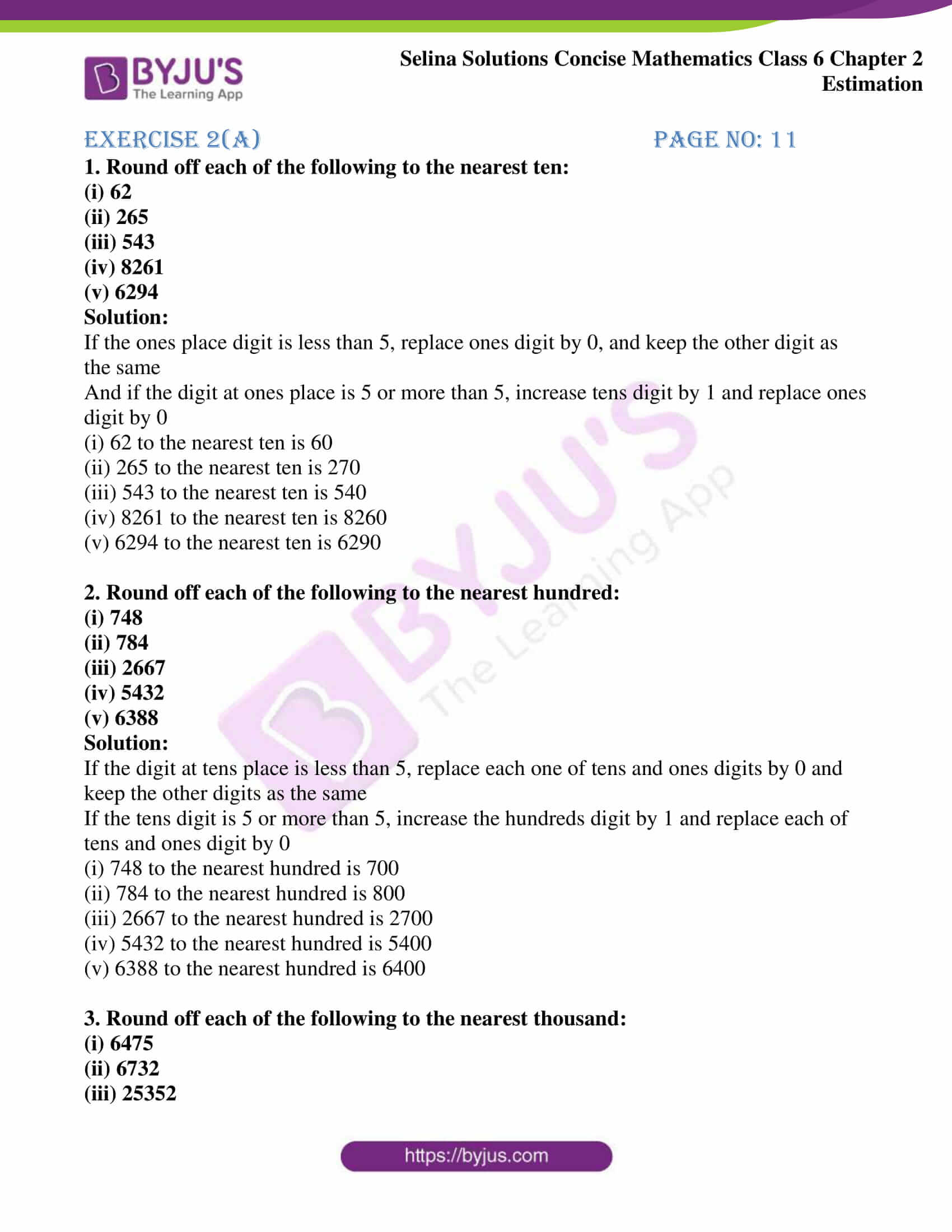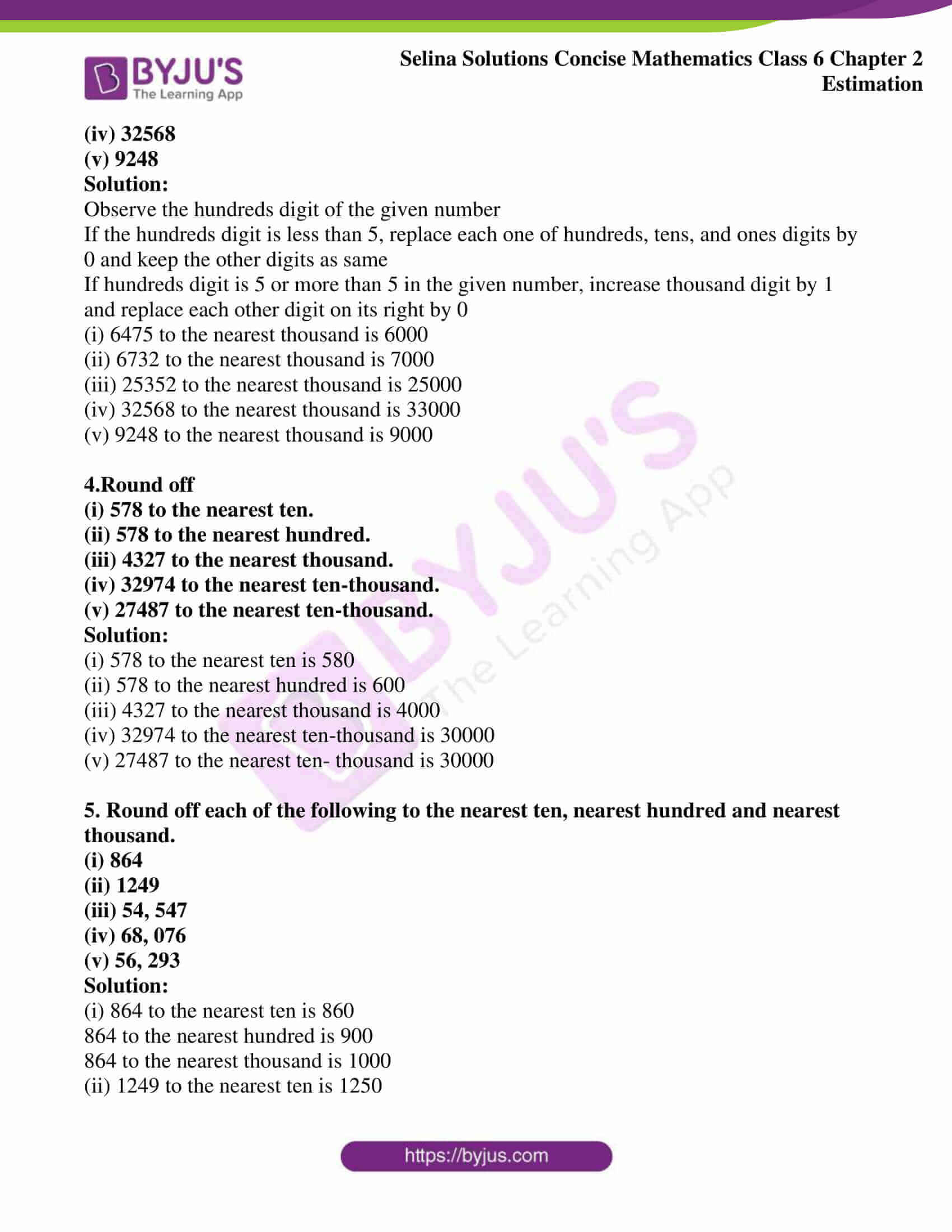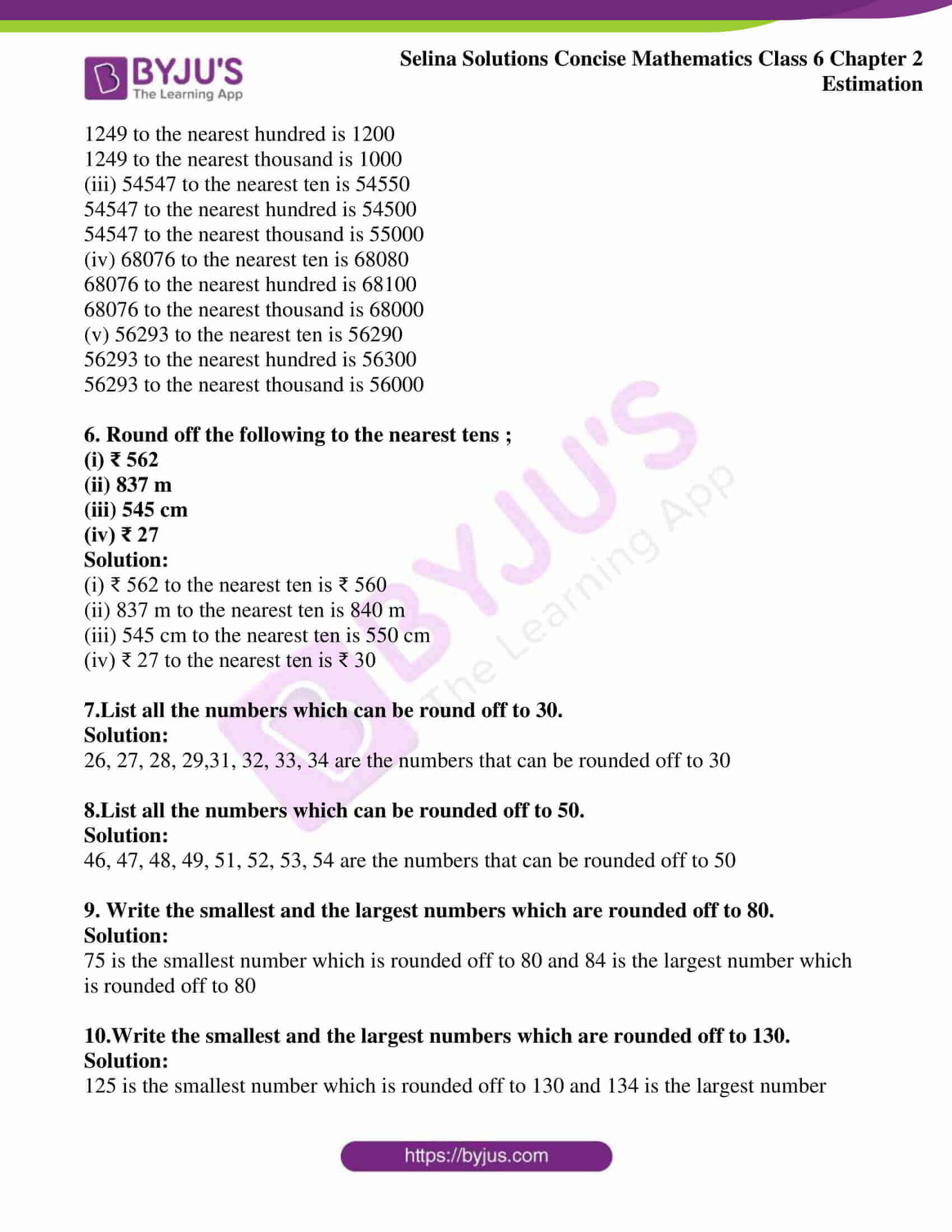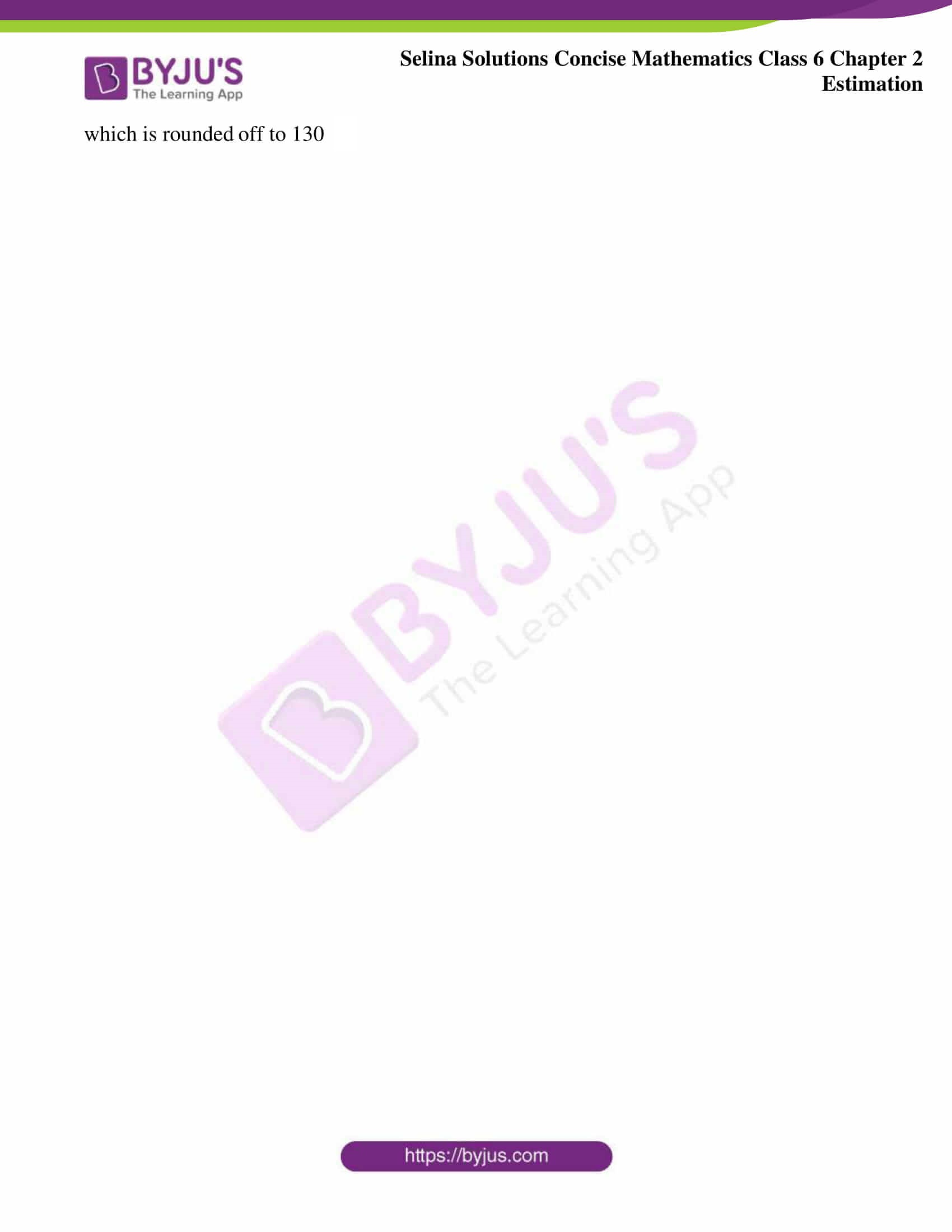# Selina Solutions Concise Mathematics Class 6 Chapter 2: Estimation Exercise 2(A)

Mathematics plays a vital role in the life of students in boosting their confidence at the time of examination. Rounding off a number is the main topic explained under this exercise. Students who want to improve their skills in the subject, are advised to follow the Selina Solutions Concise Mathematics Class 6 Chapter 2 Estimation Exercise 2(A) PDF. These Solutions, which are well structured by subject experts, help students in enhancing their performance in the examination. Download the PDF of Selina Solutions Concise Mathematics Class 6 Chapter 2 Estimation Exercise 2(A) PDF, from the links provided here.

## Selina Solutions Concise Mathematics Class 6 Chapter 2 Estimation Exercise 2(A) Download PDF### Access another exercise of Selina Solutions Concise Mathematics Class 6 Chapter 2 Estimation

Exercise 2(B) Solutions

### Access Selina Solutions Concise Mathematics Class 6 Chapter 2: Estimation Exercise 2(A)

#### Exercise 2(A) page no: 11

1. Round off each of the following to the nearest ten:

(i) 62

(ii) 265

(iii) 543

(iv) 8261

(v) 6294

Solution:

If the ones place digit is less than 5, replace ones digit by 0, and keep the other digit as the same

And if the digit at ones place is 5 or more than 5, increase tens digit by 1 and replace ones digit by 0

(i) 62 to the nearest ten is 60

(ii) 265 to the nearest ten is 270

(iii) 543 to the nearest ten is 540

(iv) 8261 to the nearest ten is 8260

(v) 6294 to the nearest ten is 6290

2. Round off each of the following to the nearest hundred:

(i) 748

(ii) 784

(iii) 2667

(iv) 5432

(v) 6388

Solution:

If the digit at tens place is less than 5, replace each one of tens and ones digits by 0 and keep the other digits as the same

If the tens digit is 5 or more than 5, increase the hundreds digit by 1 and replace each of tens and ones digit by 0

(i) 748 to the nearest hundred is 700

(ii) 784 to the nearest hundred is 800

(iii) 2667 to the nearest hundred is 2700

(iv) 5432 to the nearest hundred is 5400

(v) 6388 to the nearest hundred is 6400

3. Round off each of the following to the nearest thousand:
(i) 6475
(ii) 6732
(iii) 25352
(iv) 32568
(v) 9248

Solution:

Observe the hundreds digit of the given number

If the hundreds digit is less than 5, replace each one of hundreds, tens, and ones digits by 0 and keep the other digits as same

If hundreds digit is 5 or more than 5 in the given number, increase thousand digit by 1 and replace each other digit on its right by 0

(i) 6475 to the nearest thousand is 6000

(ii) 6732 to the nearest thousand is 7000

(iii) 25352 to the nearest thousand is 25000

(iv) 32568 to the nearest thousand is 33000

(v) 9248 to the nearest thousand is 9000

4.Round off
(i) 578 to the nearest ten.
(ii) 578 to the nearest hundred.
(iii) 4327 to the nearest thousand.
(iv) 32974 to the nearest ten-thousand.
(v) 27487 to the nearest ten-thousand.

Solution:

(i) 578 to the nearest ten is 580

(ii) 578 to the nearest hundred is 600

(iii) 4327 to the nearest thousand is 4000

(iv) 32974 to the nearest ten-thousand is 30000

(v) 27487 to the nearest ten- thousand is 30000

5. Round off each of the following to the nearest ten, nearest hundred and nearest thousand.
(i) 864
(ii) 1249
(iii) 54, 547
(iv) 68, 076
(v) 56, 293

Solution:

(i) 864 to the nearest ten is 860

864 to the nearest hundred is 900

864 to the nearest thousand is 1000

(ii) 1249 to the nearest ten is 1250

1249 to the nearest hundred is 1200

1249 to the nearest thousand is 1000

(iii) 54547 to the nearest ten is 54550

54547 to the nearest hundred is 54500

54547 to the nearest thousand is 55000

(iv) 68076 to the nearest ten is 68080

68076 to the nearest hundred is 68100

68076 to the nearest thousand is 68000

(v) 56293 to the nearest ten is 56290

56293 to the nearest hundred is 56300

56293 to the nearest thousand is 56000

6. Round off the following to the nearest tens ;
(i) ₹ 562
(ii) 837 m
(iii) 545 cm
(iv) ₹ 27

Solution:

(i) ₹ 562 to the nearest ten is ₹ 560

(ii) 837 m to the nearest ten is 840 m

(iii) 545 cm to the nearest ten is 550 cm

(iv) ₹ 27 to the nearest ten is ₹ 30

7.List all the numbers which can be round off to 30.

Solution:

26, 27, 28, 29,31, 32, 33, 34 are the numbers that can be rounded off to 30

8.List all the numbers which can be rounded off to 50.
Solution:

46, 47, 48, 49, 51, 52, 53, 54 are the numbers that can be rounded off to 50

9. Write the smallest and the largest numbers which are rounded off to 80.

Solution:

75 is the smallest number which is rounded off to 80 and 84 is the largest number which is rounded off to 80

10.Write the smallest and the largest numbers which are rounded off to 130.

Solution:

125 is the smallest number which is rounded off to 130 and 134 is the largest number which is rounded off to 130